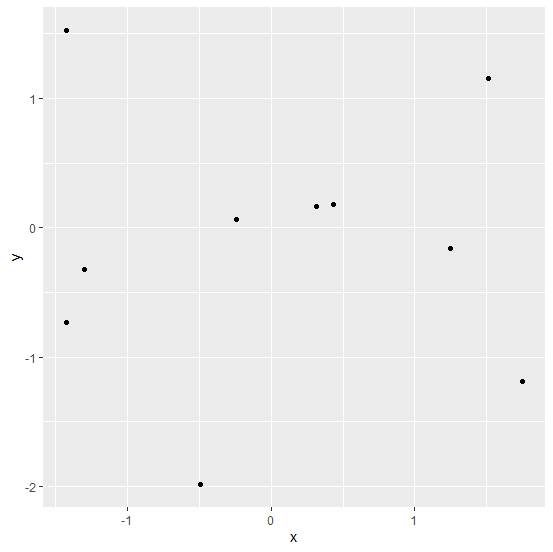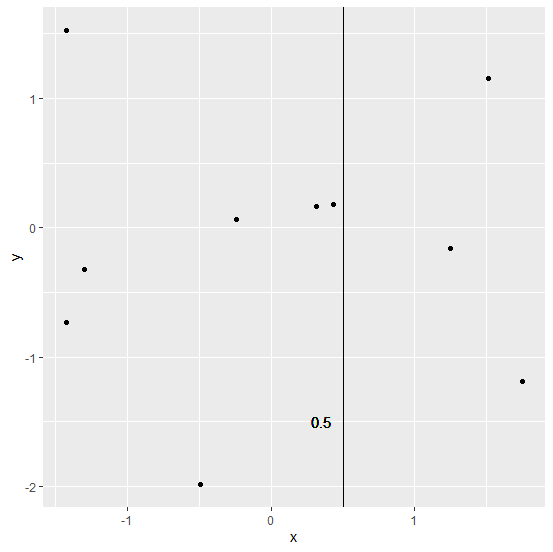# How to add a vertical line with some value on a scatterplot created by ggplot2 in R?

When we draw a scatterplot, there might be some crucial points that we would want to display, hence we create a vertical or horizontal based on our objective. These vertical or horizontal lines can be drawn by using geom_vline or geom_hline function of ggplot2 but to add some value with them we can use geom_text function.

## Example

Consider the below data frame −

Live Demo

> x<-rnorm(10)
> y<-rnorm(10,0.5)
> df<-data.frame(x,y)
> df

## Output

      x          y
1 1.2474363 -0.15892165
2 1.7511870 -1.18938250
3 -1.3001612 -0.32313571
4 -1.4220049 1.52915756
5 0.4355646 0.18282983
6 0.3128323 0.16467130
7 1.5099580 1.15199751
8 -0.4907705 -1.98635182
9 -1.4249190 -0.73298079
10 -0.2409907 0.06120644

## Example

Loading ggplot2 package and creating a scatterplot between x and y −

> library(ggplot2)
> ggplot(df,aes(x,y))+geom_point()

## Output## Example

Creating a scatterplot between x and y with a vertical line showing a value −

> ggplot(df,aes(x,y))+geom_point()+geom_vline(aes(xintercept=0.5))+
+ geom_text(aes(0,-1.5,label=0.5,hjust=-2))

## Output